地球物理学报2014, Vol. 57Issue (3): 906-917PDF

Numerical dispersion and wave-field simulation of the Runge-Kutta discontinuous Galerkin method
HE Xi-Jun, YANG Ding-Hui, WU Hao
Department of Mathematical Sciences, Tsinghua University, Beijing 100084, China
Abstract: Numerical solving of wave equations is the key point of the forward wave field simulation, seismic migration and the inversion imaging. In this paper, we study the numerical dispersion of the Runge-Kutta discontinuous Galerkin (RKDG) method for solving 2-D acoustic equations, and compare it with the ONAD method, Lax-Wendroff method and the Staggered-Grid method. Our theoretical analysis and numerical tests show that the RKDG method and the ONAD method can suppress the numerical dispersion more effectively. Especially for the three-order RKDG method, when the space step is half of the wavelength, the maximum of the numerical dispersive error will not exceed 1.67%. We also verify our conclusions by wave-field simulations.
Key words: Runge-Kutta discontinuous Galerkin method     Numerical dispersion     Wave-field simulation

1 引言

2 RKDG方法及其频散分析
2.1 RKDG方法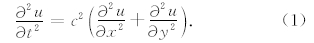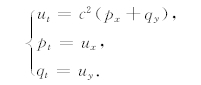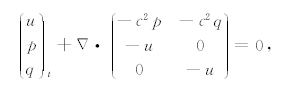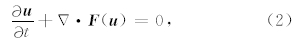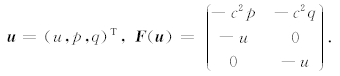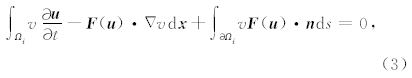∂Ωi表示区域Ωi的边界， n 表示边界∂Ωi处的单位外法向量.由于 u 在∂Ωi处不一定是连续的，所以 (3)式中的 F ( u )· n 没有定义，因此需将通量 F ( u )· n 替换为数值通量：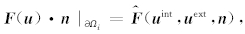其中 u int表示 u 在区域Ωi内部逼近∂Ωi处的值， u ext表示 u 在区域Ωi外部逼近∂Ωi处的值.这里采用一种 常用通量，即局部Lax-Friedrichs通量，其表达式为：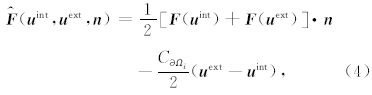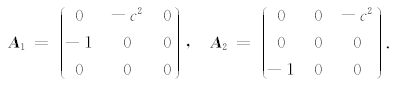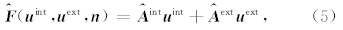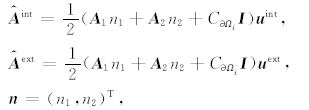I 表示单位矩阵.将 u 与v限制在试验函数空间Vh，则可得到如下公式：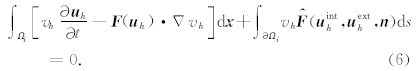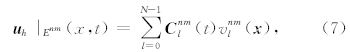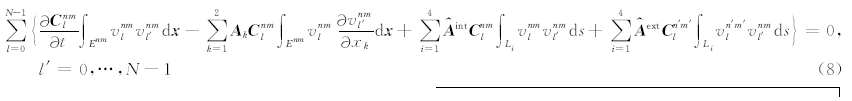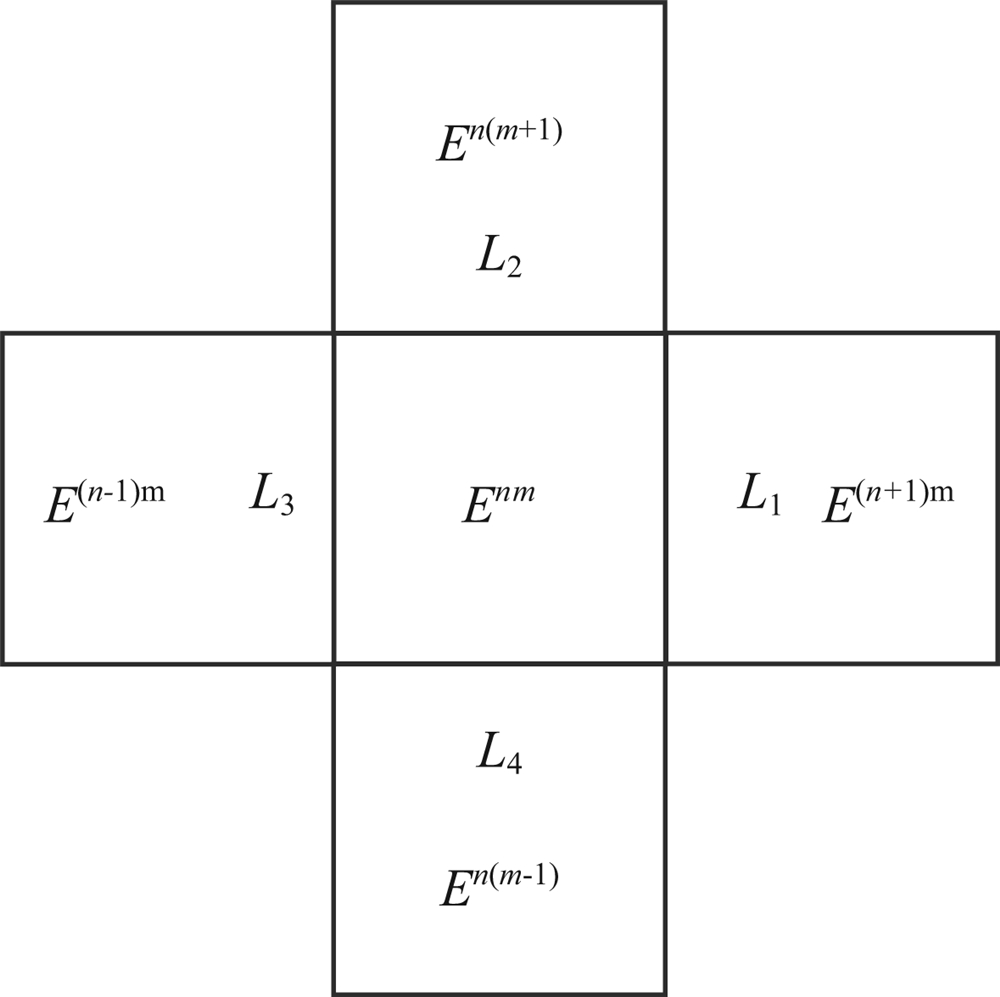图1 单元Enm及其相邻单元，以及与相邻单元相交的边界示意图 Fig.1 Schematic diagram of finite element Enm, neighboring elements and boundaries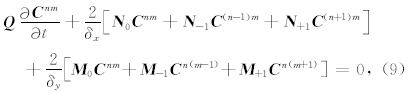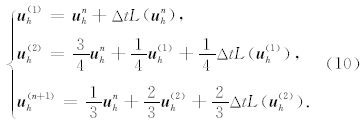2.2 RKDG的数值频散分析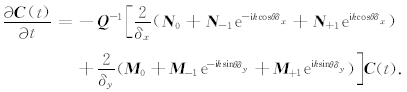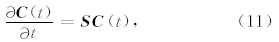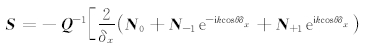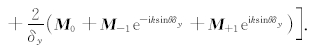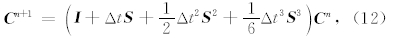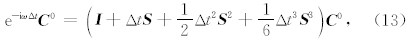3 几种不同方法的数值频散比较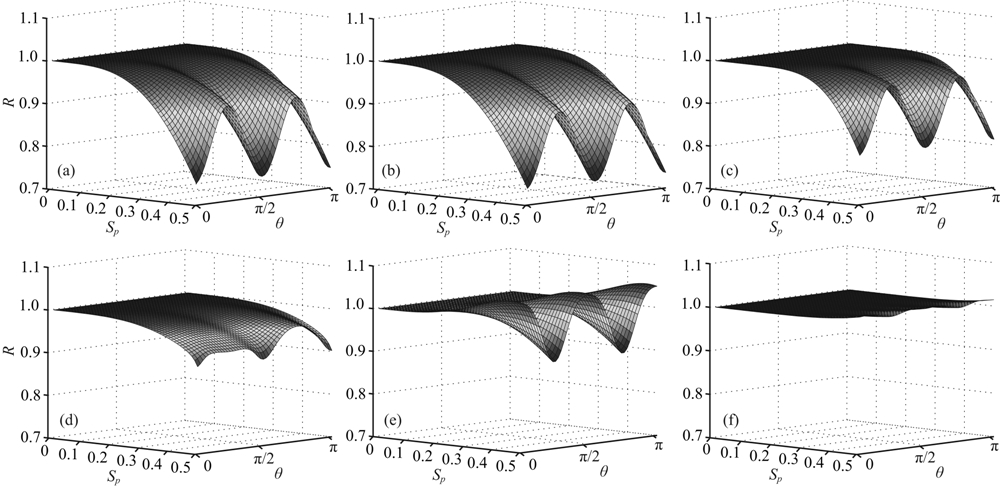图2 几种不同方法的数值频散R随采样率Sp和波传播方向θ变化的比较 (a) 4阶SG方法；(b) 4阶LWC方法；(c) 8阶LWC方法；(d) ONAD方法；(e) 2阶RKDG方法；(f) 3阶RKDG方法. Fig.2 Comparison of numerical dispersion R changing with different propagation direction θ and different sampling rate Sp for different methods (a) Fourth-order SG method; (b) Fourth-order LWC method; (c) Eighth-order LWC method; (d) ONAD method; (e) Second-order RKDG method; (f) Third-order RKDG method.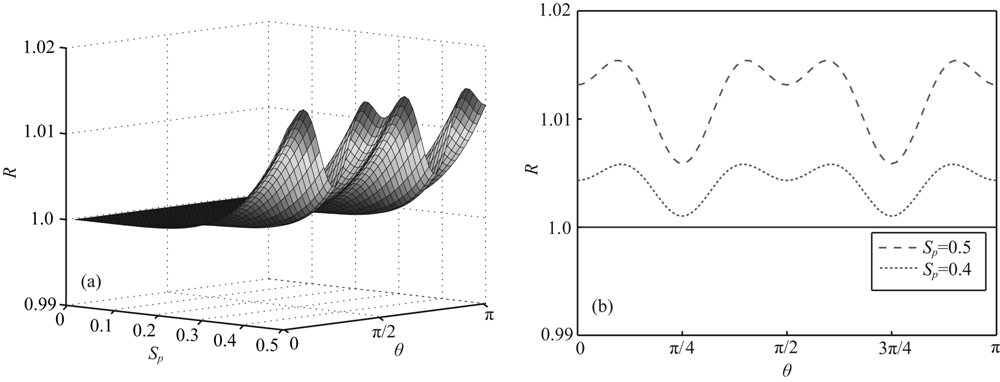图3 (a)是3阶RKDG方法的数值频散R随采样率Sp和波传播方向θ变化的情况(此图对应图2f的放大图)， (b)是在给定采样率为0.5和0.4的情况下，3阶RKDG方法的数值频散R随波传播方向θ的变化 Fig.3 (a) Numerical dispersion R for different propagation directions (θ) and different sampling rates (Sp) for the third- order RKDG method, which is the clearer version of Fig.2f; (b)Numerical dispersion R for different propagation directions θ for the third-order RKDG method with sampling rate Sp=0.5 and Sp=0.4, respectively表1 不同库朗数条件下不同数值方法的最大数值频散误差(%) Table 1 Maximum numerical dispersive errors of different numerical methods for different Courant numbers (%)

4 效率比较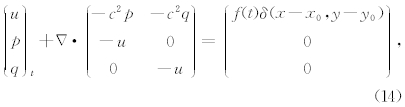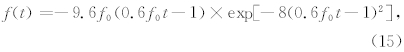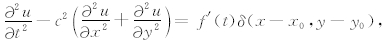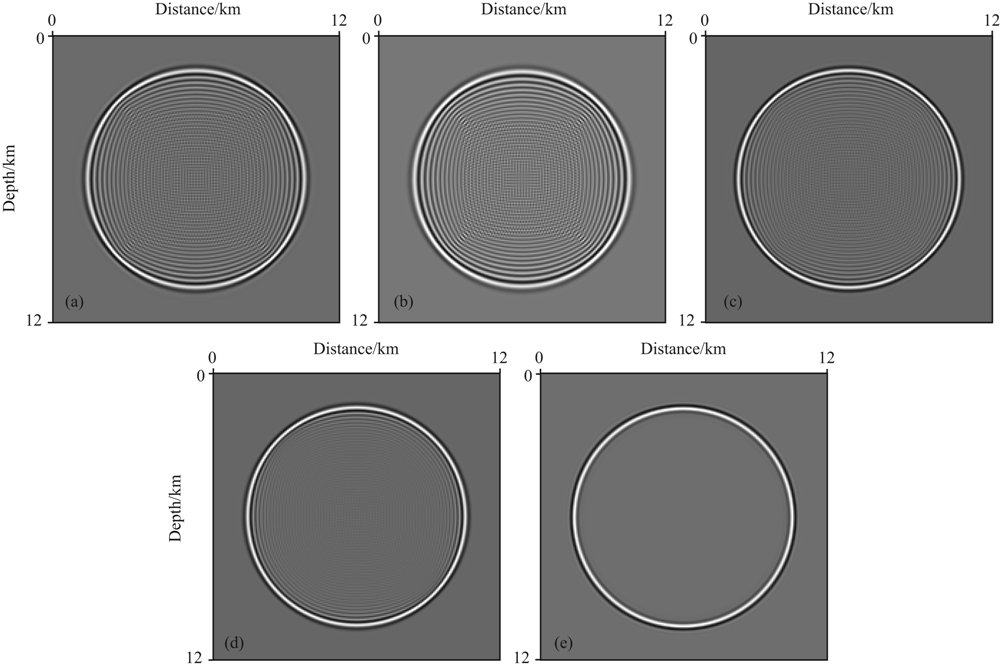图4 粗网格(Δx=Δy=60 m)条件下T=1.2 s时刻的波场瞬时快照 (a)4阶SG方法；(b)4阶LWC方法；(c)8阶LWC方法；(d)ONAD方法；(e) 3阶RKDG方法. Fig.4 Snapshots of acoustic wave fields at time T=1.2 s on the coarse grid (Δx=Δy=60 m) Generated by the (a) fourth-order SG method, (b) fourth-order LWC method, (c) eighth-order LWC method, (d) ONAD method, and (e) third-order RKDG method.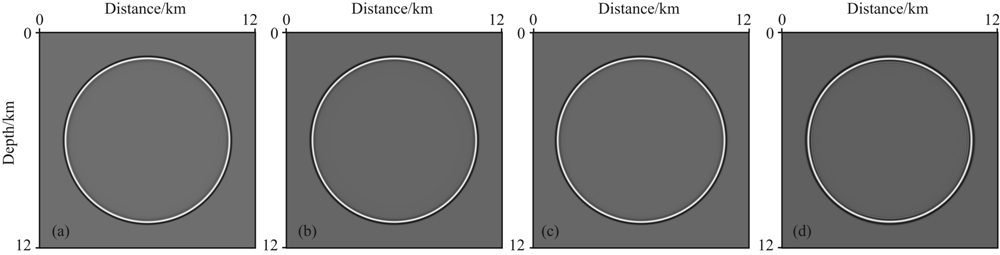图5 无可见数值频散条件下T=1.2 s时刻的波场快照 (a)4阶SG方法(Δx=Δy=15 m)；(b)4阶LWC方法(Δx=Δy=15 m)； (c)8阶LWC方法(Δx=Δy=20 m)；(d)ONAD方法(Δx=Δy=40 m). Fig.5 Snapshots of acoustic wave fields at time T=1.2 s Generated by the (a) fourth-order SG method (Δx=Δy=15 m), (b) fourth-order LWC method (Δx=Δy=15 m), (c) eighth-order LWC method (Δx=Δy=20 m), and (d) ONAD method (Δx=Δy=40 m), respectively.表2 不同数值方法的计算CPU计时和存储量比较 Table 2 Comparison of CPU time and computer memory for different numerical methods
5 波场模拟

5.1 双层介质模型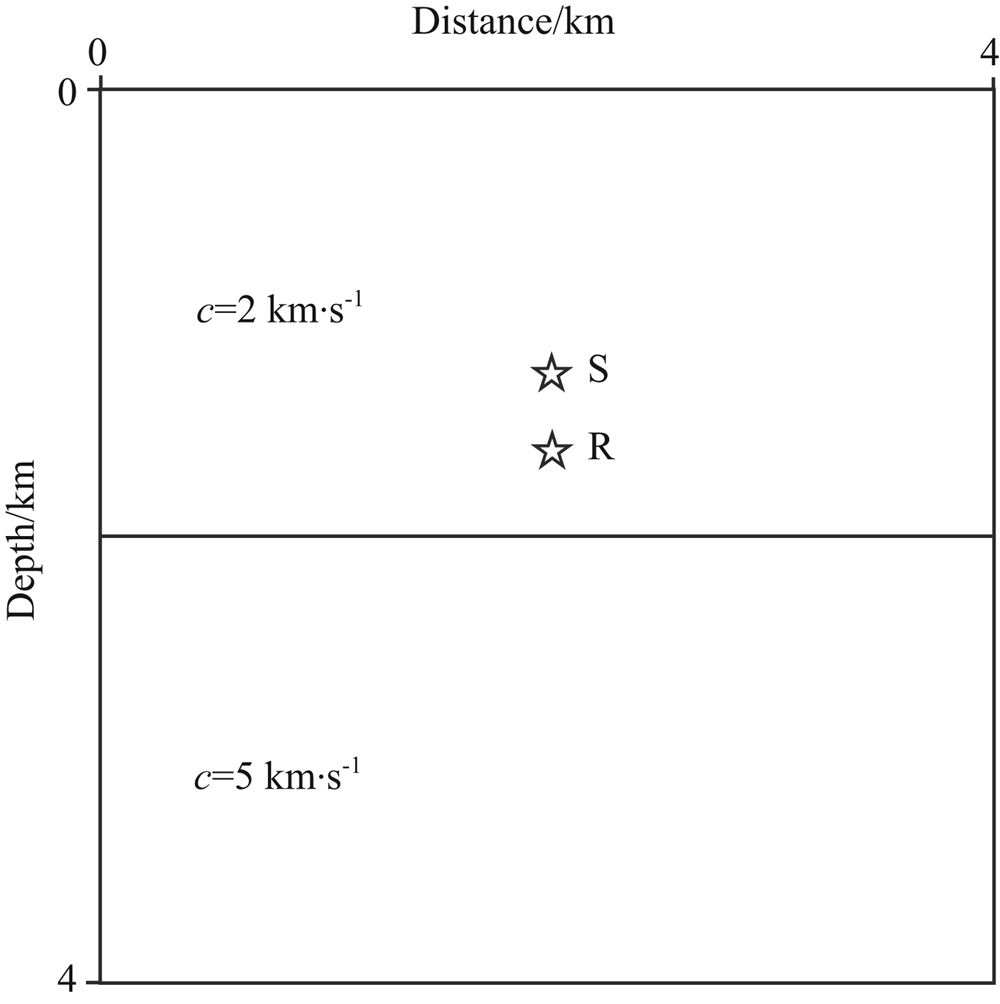图6 双层介质模型，在深度z=2 km处有一强间断 震源S位于z=1.6 km处，接收点R位于z=1.8 km处. Fig.6 Two-layer medium model with a strong discontinuity at depth of z=2 km The explosive source denoted by S is located at z=1.6 km, while the receiver R is located at z=1.8 km.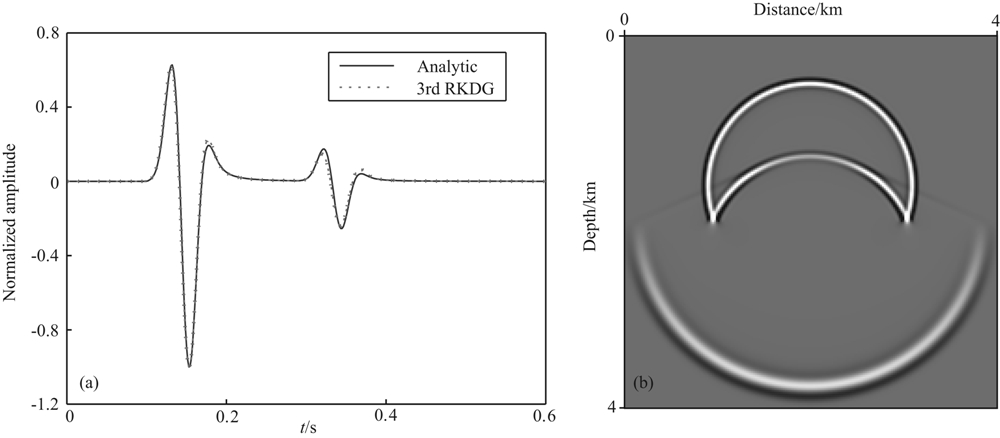图7 对于双层介质模型，3阶RKDG方法在空间步长Δx=Δy=20 m，T=0.6 s 时刻的(a)波形记录(其中实线为解析解，虚线为数值解)和(b)波场快照 Fig.7 Waveforms (a) (where the solid line denotes the analytic solution and the dotted line denotes the numerical solution) and the snapshot of seismic wave fields (b) on the grid Δx=Δy=20 m, at time T=0.6 s, generated by the third-order RKDG method for the two-layer medium model
5.2 Marmousi模型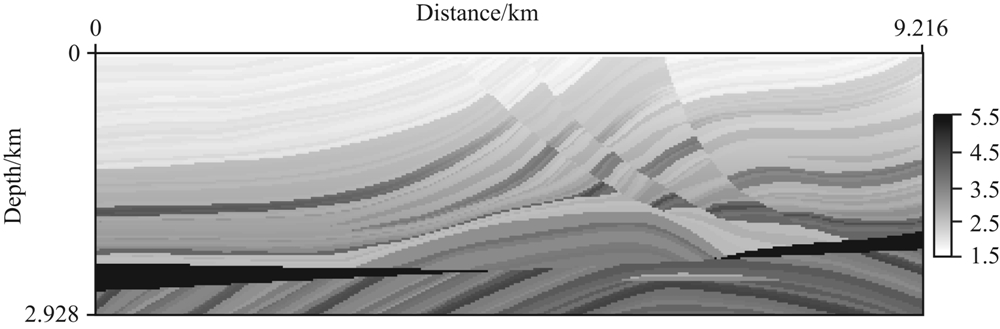图8 Marmousi速度模型 Fig.8 Marmousi model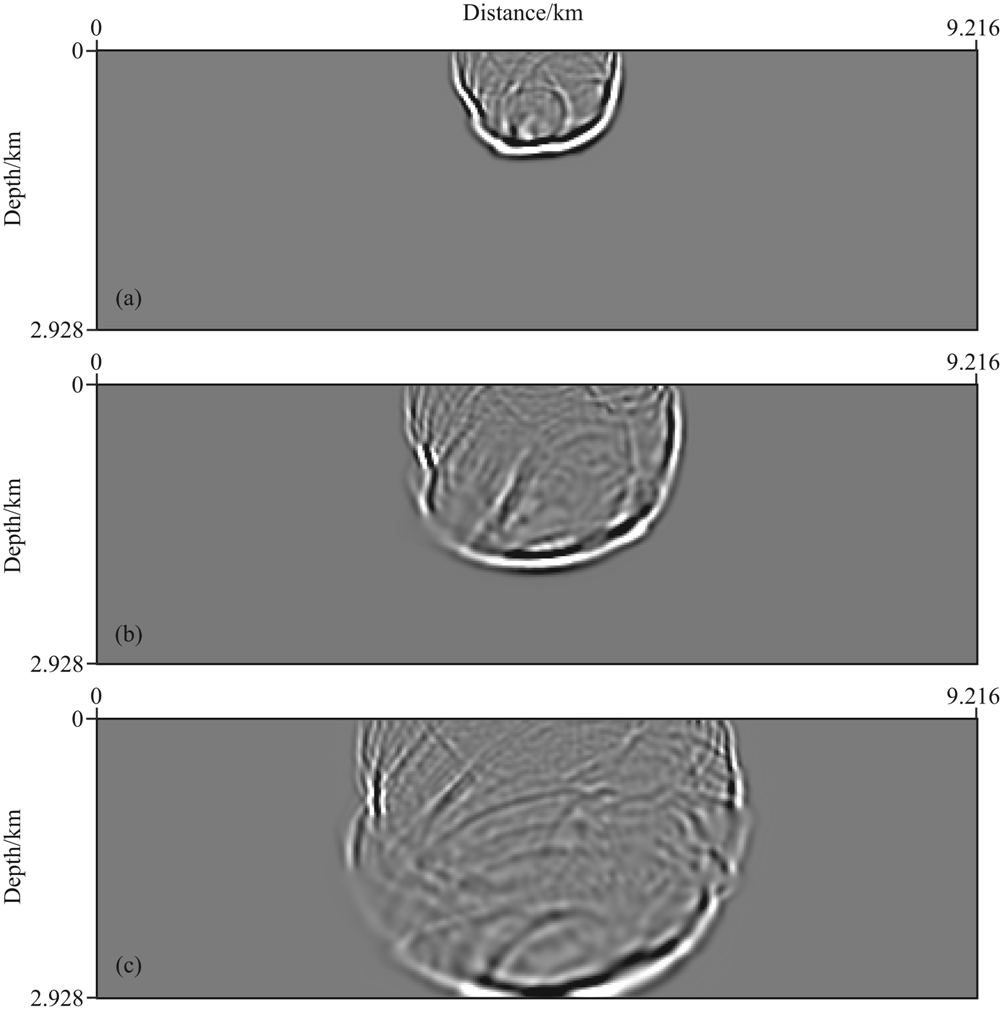图9 对于Marmousi模型，利用3阶RKDG方法模拟声波所得到的不同时刻的波场快照 (a) T=0.6 s; (b) T=0.9 s; (c) T=1.2 s. Fig.9 Snapshots at different times generated by the third-order RKDG method for the Marmousi model
6 结论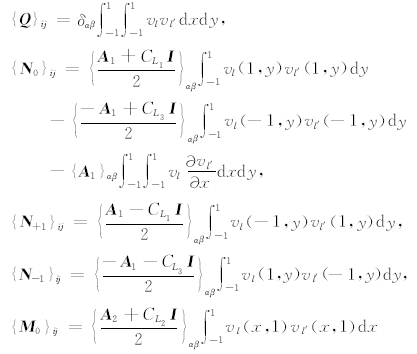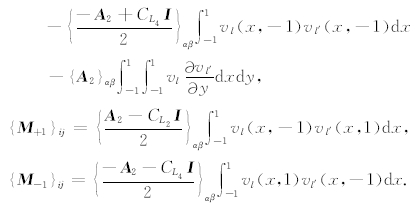i=ml′+α, j=ml+β;α,β=0,1,…,m－1;l′,l=0,1,…,N－1. N为单元上基函数的个数，m为 u 中未知量的个数.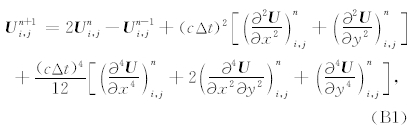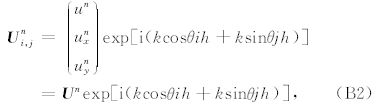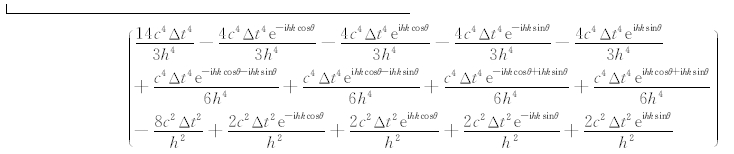再令 U n= U 0e－iωnΔt并代入(B3)式，可得 (e－iωΔt－2+eiωΔt) U 0= HU 0.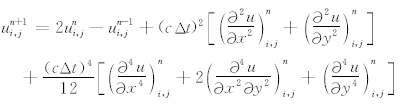e1=－30+16d1－d2,

e2=152－125d1+20d2－d3+64f1,1－2f1,2,

dn=cos(2πnSpcosθ)+cos(2πnSpsinθ),n=1,2,3

fm,n=cos(2πmSpcosθ)+cos(2πnSpsinθ),m=1,2.

SG交错方法最初由Virieux(19841986)提出，最初针对速度应力方程，后来推广到位移应力方程.其中的文中给出了详细的格式说明与频散分析.我们将格式列出如下：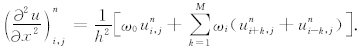其中u定义在整网格点(i,j)上，τ定义在整网格点(i+1/2,j)上，η定义在整网格点(i,j+1/2)上，a=－1/24，b=9/8.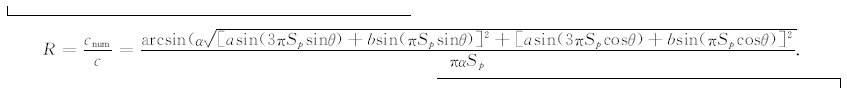Arnold D N, Brezzi F, Cockburn B, et al. 2002. Unified analysis of Discontinuous Galerkin Methods for elliptic problems. SIAM Journal on Numerical Analysis, 39(5): 1749-1779, doi: 10.1137/S0036142901384162.  Chen K H. 1984. Propagating numerical model of elastic wave in anisotropic in homogeneous media-finite element method. The 54th SEG Annual meeting Expanded Abstracts, 631-632.  Cohen G C. 2002. Higher-order Numerical Methods for Transient Wave Equations. Springer.  Cockburn B, Shu C W. 1989. TVB Runge-Kutta local projection discontinuous Galerkin finite element method for conservation laws II: General framework. Mathematics of Computation, 52(186): 411-435.  Cockburn B, Shu C W. 1998. The Runge-Kutta discontinuous Galerkin method for conservation laws V: Multidimensional systems. Journal of Computational Physics, 141(2): 199-224, doi: 10.1006/jcph.1998.5892.  Cockburn B, Shu C W. 2001. Runge-Kutta discontinuous Galerkin methods for Convection-Dominated problems. Journal of Scientific Computing, 16(3): 173-261, doi: 10.1023/ A:1012873910884.  Dablain M A. 1986. The application of high-order differencing to scalar wave equation. Geophysics, 51(1): 54-66, doi: 10.1190/1.1442040.  De Basabe J D, Sen M K. 2007. Grid dispersion and stability criteria of some common finite-element methods for acoustic and elastic wave equations. Geophysics, 72(6): T81-T95, doi: 10.1190/1.2785046.  De Basabe J D, Sen M K, Wheeler M F. 2008. The interior penalty discontinuous Galerkin method for elastic wave propagation: Grid dispersion. Geophysical Journal International, 175(1): 83-93, doi: 10.1111/j.1365-246X.2008.03915.x.  Dong L G, Ma Z T, Cao J Z, et al. 2000. A staggered-grid high-order difference method of one-order elastic wave equation. Chinese J. Geophys. (in Chinese), 43(3): 411-419.  Dumbser M, Käser M. 2006. An arbitrary high order discontinuous Galerkin method for elastic waves on unstructured meshes—II: The three-dimensional case. Geophysical Journal International, 167(1): 319-336, doi: 10.1111/j.1365-246X.2006.03120.x.  Fei T, Larner K. 1995. Elimination of numerical dispersion in finite difference modeling and migration by flux-corrected transport. Geophysics, 60: 1830-1842, doi: 10.1190/1.1443915.  Hesthaven J S, Warburton T. 2008. Nodal Discontinuous Galerkin Methods: Algorithms, Analysis, and Applications. Springer.  Hu F Q, Hussaini M Y, Rasitarinera P. 1999. An analysis of the discontinuous Galerkin method for wave propagation problems. Journal of Computational Physics, 151(2): 921-946, doi: 10.1006/jcph.1999.6227.  Käser M, Dumbser M. 2006. An arbitrary high-order discontinuous Galerkin method for elastic waves on unstructured meshes—I: The two-dimensional isotropic case with external source terms. Geophysical Journal International, 166(2): 855-877, doi: 10.1111/j.1365-246X.2006.03051.x.  Kelly K R, Wave R W, Treitel S, et al. 1976. Synthetic seismograms: a finite-difference approach. Geophysics, 41(1): 2-27, doi: 10.1190/1.1440605.  Komatitsch D, Vilotte J P. 1998. The spectral element method: an efficient tool to simulate the seismic responses of 2D and 3D geological structures. Bulletin of the Seismological Society of America, 88(2): 368-392.  Kondoh Y, Hosaka Y, Ishii K. 1994. Kernel optimum nearly-analytical discretization algorithm applied to parabolic and hyperbolic equations. Computers & Mathematics with Applications, 27(3): 59-90, doi: 10.1016/0898-1221(94)90047-7.  Ma X, Yang D H, Liu F Q. 2011. A nearly analytic symplectically partitioned Runge-Kutta method for 2-D seismic wave equations. Geophysical Journal International, 187(1): 480-496, doi: 10.1111/j.1365-246X.2011.05158.x.  Ma X, Yang D H, Zhang J H. 2010. Symplectic partitioned Runge-Kutta method for solving the acoustic wave equation. Chinese J. Geophys. (in Chinese), 53(8): 1993-2003, doi: 10.3969/j.issn.0001-5733.2010.08.026.  Moczo P, Kristek J, Halada L. 2000. 3D 4th-order staggered-grid finite-difference schemes: stability and grid dispersion. Bulletin of the Seismological Society of America, 2000, 90(3): 587-603, doi: 10.1785/0119990119.  Reed W H, Hill T R. 1973. Triangular mesh methods for the neutron transport equation. Los Alamos Scientific Laboratory Report. LA-UR-73-479.  Versteeg R J, Grau G. 1991. The Marmousi Experience. Proc. EAGE Workshop on Practical Aspects of Seismic Data Inversion, Eur. Assoc. Explor. Geophysicists, Zeist.  Virieux J. 1984. SH-wave propagation in heterogeneous media: velocity-stress finite-difference method. Geophysics, 49(11): 1933-1957, doi: 10.1190/1.1441605.  Virieux J. 1986. P-SV wave propagation in heterogeneous media: velocity-stress finite-difference method. Geophysics, 51(4): 889-901, doi: 10.1190/1.1442147.  Wang L, Yang D H, Deng X Y. 2009. A WNAD method for seismic stress-field modeling in heterogeneous media. Chinese J. Geophys. (in Chinese), 52(6): 1526-1535, doi: 10.3969/j.issn.0001-5733.2009.06.014.  Yang D H, Peng J M, Lu M, et al. 2006. Optimal nearly-analytic discrete approximation to the scalar wave equation. Bulletin of the Seismological Society of America, 96(3): 1114-1130, doi: 10.1785/0120050080.  Yang D H, Song G J, Chen S, et al. 2007. An improved nearly analytical discrete method: an efficient tool to simulate the seismic response of 2-D porous structures. Journal of Geophysics and Engineering, 4(1): 40-52, doi: 10.1088/1742-2132/4/1/006.  Yang D H, Song G J, Hua B L, et al. 2010. Simulation of acoustic wavefields in heterogeneous media: A robust method for automatic suppression of numerical dispersion. Geophysics, 75(3): T99-T110, doi: 10.1190/1.3428483.  Yang D H, Teng J W, Zhang Z J, et al. 2003. A nearly-analytic discrete method for acoustic and elastic wave equations in anisotropic media. Bulletin of the Seismological Society of America, 93(2): 882-890, doi: 10.1785/0120020125.  Zheng H S, Zhang Z J, Liu E. 2006. Non-linear seismic wave propagation in anisotropic media using flux-corrected transport technique. Geophysical Journal International, 165(3): 943-956, doi: 10.1111/j.1365-246X.2006.02966.x.  董良国, 马在田, 曹景忠等. 2000. 一阶弹性波方程交错网格高阶差分解法. 地球物理学报, 43(3): 411-419.  马啸, 杨顶辉, 张锦华. 2010. 求解声波方程的辛可分Runge-Kutta方法. 地球物理学报, 53(8): 1993-2003, 10.3969/j.issn.0001-5733.2010.08.026.  王磊, 杨顶辉, 邓小英. 2009. 非均匀介质中地震波应力场的WNAD方法及其数值模拟. 地球物理学报, 52(6):1526-1535, doi: 10.3969/j.issn.0001-5733.2009.06.014.